06-1350/Class Notes for Thursday November 16

(diff) ← Older revision | Latest revision (diff) | Newer revision → (diff)

Today's Agenda

Formulas are a Chore (Bore?)

• Sweeping clean a tree and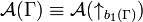${\mathcal A}(\Gamma)\equiv{\mathcal A}(\uparrow_{b_1(\Gamma)})$.
•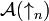${\mathcal A}(\uparrow_n)$ is a VS-algebra (see more at VS, TS and TG Algebras).
• In the coordinates above, write the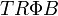$TR\Phi B$ relations in various algebraic notations.
• R4: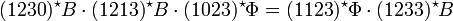$(1230)^\star B\cdot(1213)^\star B\cdot(1023)^\star\Phi=(1123)^\star\Phi\cdot(1233)^\star B$ or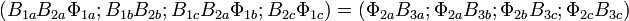$(B_{1a}B_{2a}\Phi_{1a}; B_{1b}B_{2b}; B_{1c}B_{2a}\Phi_{1b}; B_{2c}\Phi_{1c}) = (\Phi_{2a}B_{3a}; \Phi_{2a}B_{3b}; \Phi_{2b}B_{3c}; \Phi_{2c}B_{3c})$.
• R3: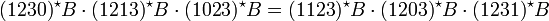$(1230)^\star B\cdot(1213)^\star B\cdot(1023)^\star B = (1123)^\star B\cdot(1203)^\star B\cdot(1231)^\star B$ or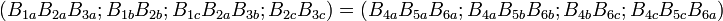$(B_{1a}B_{2a}B_{3a}; B_{1b}B_{2b}; B_{1c}B_{2a}B_{3b}; B_{2c}B_{3c}) = (B_{4a}B_{5a}B_{6a}; B_{4a}B_{5b}B_{6b}; B_{4b}B_{6c}; B_{4c}B_{5c}B_{6a})$.
• R2: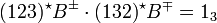$(123)^\star B^\pm\cdot(132)^\star B^\mp=1_3$ or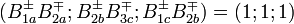$(B^\pm_{1a}B^\mp_{2a}; B^\pm_{2b}B^\mp_{3c}; B^\pm_{1c}B^\mp_{2b}) = (1;1;1)$.
• R1: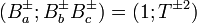$(B^\pm_a; B^\pm_bB^\pm_c)=(1;T^{\pm 2})$.
• But for now, skip the writing of the following relations:
• Symmetry of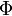$\Phi$ and of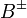$B^{\pm}$.
•$u$,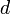$d$ and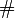$\#$
• Idempotence for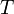$T$,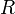$R$,$\Phi$ and$B^{\pm}$.
•$B^{\pm}$ in terms of$\Phi$ and$R$ and$R$ in terms of$T$.

Exponentiation is a Miracle

• Description of the problem.
• Beads and strands.
• The perturbative approach, linearization.
• The syzygies: relations between the errors.
• The Hochschild complex and homology.